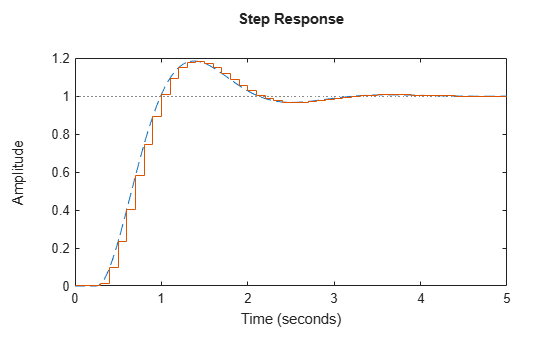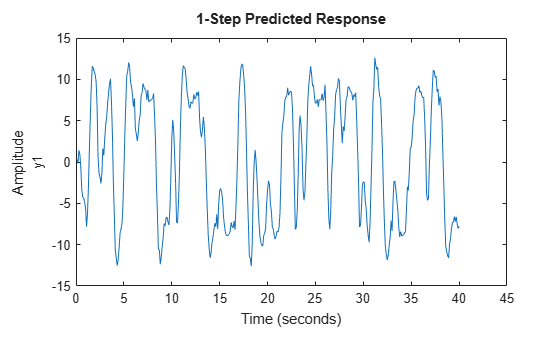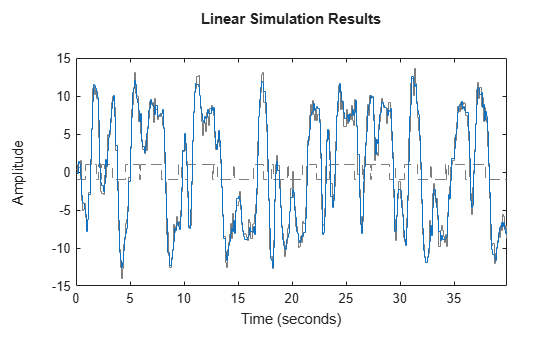c2d

Convert model from continuous to discrete time

Description

example

sysd = c2d(sysc,Ts) discretizes the continuous-time dynamic system model sysc using zero-order hold on the inputs and a sample time of Ts.

example

sysd = c2d(sysc,Ts,method) specifies the discretization method.

example

sysd = c2d(sysc,Ts,opts) specifies additional options for the discretization.

[sysd,G] = c2d(___), where sysc is a state-space model, returns a matrix, G that maps the continuous initial conditions x0 and u0 of the state-space model to the discrete-time initial state vector x.

Examples

collapse all

Discretize the following continuous-time transfer function:

$H\left(s\right)={e}^{-0.3s}\frac{s-1}{{s}^{2}+4s+5}.$

This system has an input delay of 0.3 s. Discretize the system using the triangle (first-order-hold) approximation with sample time Ts = 0.1 s.

H = tf([1 -1],[1 4 5],'InputDelay', 0.3);
Hd = c2d(H,0.1,'foh');

Compare the step responses of the continuous-time and discretized systems.

step(H,'-',Hd,'--')Discretize the following delayed transfer function using zero-order hold on the input, and a 10-Hz sampling rate.

$H\left(s\right)={e}^{-0.25s}\frac{10}{{s}^{2}+3s+10}.$

h = tf(10,[1 3 10],'IODelay',0.25);
hd = c2d(h,0.1)
hd =

0.01187 z^2 + 0.06408 z + 0.009721
z^(-3) * ----------------------------------
z^2 - 1.655 z + 0.7408

Sample time: 0.1 seconds
Discrete-time transfer function.

In this example, the discretized model hd has a delay of three sampling periods. The discretization algorithm absorbs the residual half-period delay into the coefficients of hd.

Compare the step responses of the continuous-time and discretized models.

step(h,'--',hd,'-')Create a continuous-time state-space model with two states and an input delay.

sys = ss(tf([1,2],[1,4,2]));
sys.InputDelay = 2.7
sys =

A =
x1  x2
x1  -4  -2
x2   1   0

B =
u1
x1   2
x2   0

C =
x1   x2
y1  0.5    1

D =
u1
y1   0

Input delays (seconds): 2.7

Continuous-time state-space model.

Discretize the model using the Tustin discretization method and a Thiran filter to model fractional delays. The sample time Ts = 1 second.

opt = c2dOptions('Method','tustin','FractDelayApproxOrder',3);
sysd1 = c2d(sys,1,opt)
sysd1 =

A =
x1         x2         x3         x4         x5
x1    -0.4286    -0.5714   -0.00265    0.06954      2.286
x2     0.2857     0.7143  -0.001325    0.03477      1.143
x3          0          0    -0.2432     0.1449    -0.1153
x4          0          0       0.25          0          0
x5          0          0          0      0.125          0

B =
u1
x1  0.002058
x2  0.001029
x3         8
x4         0
x5         0

C =
x1         x2         x3         x4         x5
y1     0.2857     0.7143  -0.001325    0.03477      1.143

D =
u1
y1  0.001029

Sample time: 1 seconds
Discrete-time state-space model.

The discretized model now contains three additional states x3, x4, and x5 corresponding to a third-order Thiran filter. Since the time delay divided by the sample time is 2.7, the third-order Thiran filter ('FractDelayApproxOrder' = 3) can approximate the entire time delay.

Estimate a continuous-time transfer function, and discretize it.

sys1c = tfest(z1,2);
sys1d = c2d(sys1c,0.1,'zoh');

Estimate a second order discrete-time transfer function.

sys2d = tfest(z1,2,'Ts',0.1);

Compare the response of the discretized continuous-time transfer function model, sys1d, and the directly estimated discrete-time model, sys2d.

compare(z1,sys1d,sys2d)The two systems are almost identical.

Discretize an identified state-space model to build a one-step ahead predictor of its response.

Create a continuous-time identified state-space model using estimation data.

sysc = ssest(z2,4);

Predict the 1-step ahead predicted response of sysc.

predict(sysc,z2)Discretize the model.

sysd = c2d(sysc,0.1,'zoh');

Build a predictor model from the discretized model, sysd.

[A,B,C,D,K] = idssdata(sysd);
Predictor = ss(A-K*C,[K B-K*D],C,[0 D],0.1);

Predictor is a two-input model which uses the measured output and input signals ([z1.y z1.u]) to compute the 1-step predicted response of sysc.

Simulate the predictor model to get the same response as the predict command.

lsim(Predictor,[z2.y,z2.u])The simulation of the predictor model gives the same response as predict(sysc,z2).

Input Arguments

collapse all

Continuous-time model, specified as a dynamic system model such as tf, ss, or zpk. sysc cannot be a frequency response data model. sysc can be a SISO or MIMO system, except that the 'matched' discretization method supports SISO systems only.

sysc can have input/output or internal time delays; however, the 'matched', 'impulse', and 'least-squares' methods do not support state-space models with internal time delays.

The following identified linear systems cannot be discretized directly:

• idgrey models whose FunctionType is 'c'. Convert to idss model first.

• idproc models. Convert to idtf or idpoly model first.

Sample time, specified as a positive scalar that represents the sampling period of the resulting discrete-time system. Ts is in TimeUnit, which is the sysc.TimeUnit property.

Discretization method, specified as one of the following values:

• 'zoh' — Zero-order hold (default). Assumes the control inputs are piecewise constant over the sample time Ts.

• 'foh' — Triangle approximation (modified first-order hold). Assumes the control inputs are piecewise linear over the sample time Ts.

• 'impulse' — Impulse invariant discretization

• 'tustin' — Bilinear (Tustin) method. To specify this method with frequency prewarping (formerly known as the 'prewarp' method), use the PrewarpFrequency option of c2dOptions.

• 'matched' — Zero-pole matching method

• 'least-squares' — Least-squares method

• 'damped' — Damped Tustin approximation based on the TRBDF2 formula for sparse models only.

For information about the algorithms for each conversion method, see Continuous-Discrete Conversion Methods.

Discretization options, specified as a c2dOptions object. For example, specify the prewarp frequency, order of the Thiran filter or discretization method as an option.

Output Arguments

collapse all

Discrete-time model, returned as a dynamic system model of the same type as the input system sysc.

When sysc is an identified (IDLTI) model, sysd:

• Includes both measured and noise components of sysc. The innovations variance λ of the continuous-time identified model sysc, stored in its NoiseVarianceproperty, is interpreted as the intensity of the spectral density of the noise spectrum. The noise variance in sysd is thus λ/Ts.

• Does not include the estimated parameter covariance of sysc. If you want to translate the covariance while discretizing the model, use translatecov.

Mapping of continuous-time initial conditions x0 and u0 of the state-space model sysc to the discrete-time initial state vector x, returned as a matrix. The mapping of initial conditions to the initial state vector is as follows:

$x\left[\text{ }0\right]=G\cdot \left[\begin{array}{c}{x}_{0}\\ {u}_{0}\end{array}\right]$

For state-space models with time delays, c2d pads the matrix G with zeroes to account for additional states introduced by discretizing those delays. See Continuous-Discrete Conversion Methods for a discussion of modeling time delays in discretized systems.